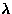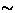Next: 6.2.4 Discussion Up: 5.2 Haldane material YBaNiO Previous: Nominally pure and the

### Charge doped systems

In the Ca doped systems, a sharp increase of the relaxation rate () was observed as the temperature approachedfrom the paramagnetic side (Fig.41). The increase of the relaxation rate probably reflects the critical slowing down of the moments, as has been observed typically in spin glass systems . In the Ca doped x=4.5% sample, the temperature dependence ofsuggests a glass temperatureK, which was below the temperature of our SQUID magnetometer.In order to investigate field fluctuations in the charge doped system, we performed longitudinal field measurements. The results are shown in Fig. 43. The zero-field (ZF) spectrum exhibited a Gaussian decay, which seemed to be the Gaussian Kubo-Toyabe function in the slow fluctuation regime (see section 3.1). However, the LF dependence of the relaxation didn't follow the predictions of the Gaussian Kubo-Toyabe theory. If the Gaussian behavior in the zero-field were due to almost static Gaussian field distribution, the relaxation should have been decoupled in a LF200 G, while in fact, the relaxation was present up to LF=2 kG. There are two unconventional behaviors presented in this result:
(1)
The weak LF dependence of the relaxation suggests persistent dynamics in the spin system, even though the temperature (T=50 mK) is well below the glass temperature ().
(2)
There has been no theories which allow the coexistence of the zero-field Gaussian decay and fast field fluctuations. In the framework of the conventional Kubo-Toyabe theory, fast fluctuation induces either an exponential function (dense spin system) or a square-root exponential function (dilute spin system), but never a Gaussian decay (see Chapter 3).
This result is further investigated in the next section.Next: 6.2.4 Discussion Up: 5.2 Haldane material YBaNiO Previous: Nominally pure and the